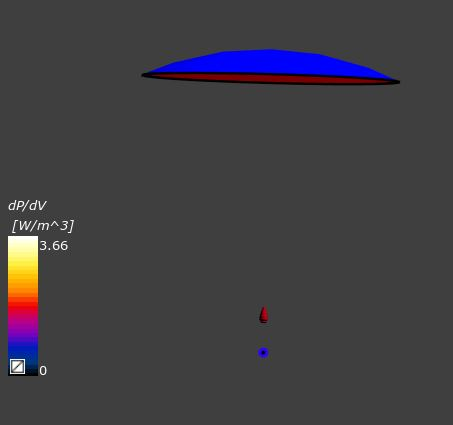# How to calculate the volmue value of deposited energy in acoustic simulation?Hi

I am doing the acoustic simulation by using SEFT.
In the post-processing step, how could I calculate the volume value of the deposited energy?